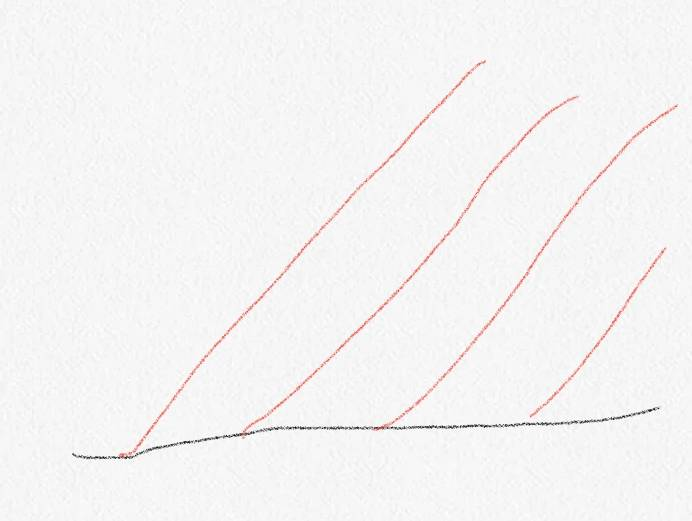# Why do higher frequency light waves bend more in a prism?

For example, why does blue light refract more than red light in a prism?

2022 Award
What does Googling this tell you? Or your textbook?

•berkeman
What does Googling this tell you? Or your textbook?
Googling it provides this answer: "Higher frequencies of light are refracted more because objects are more likely to absorb higher frequency light. This causes those frequencies of light to travel more slowly and be refracted more."
It doesn't say how though.

I already returned the textbook to the library.

2022 Award
That's half right. The "more likely to absorb" thing is a common misconception. Light in matter doesn't work that way. However, the speed change is important.

Draw a diagram with a horizontal line for the boundary between the media. Draw lines at an angle to it, representing the position of a single wave crest at a sequence of times:Apologies for the lousy sketching - drawing with my finger on my phone screen on a very cold platform. But hopefully you get the idea - the red lines are perpendicular to the incident ray and show where a wave crest is now (right hand line), and where it was one, two, and three cycles ago (the other lines). The black line is the boundary.

If you draw this with a ruler and with even spacing (say 2cm) between each red line then you can work out what happens. Say the refractive index is n=2, for easy calculation. Where could the bits of the wave crest that are in the medium be now? Well, the rightmost line isn't much help. The line next to it, however, you know that the point that just touched the boundary has traveled 2cm/n=1cm since it was drawn. So if you draw a semicircle below the boundary of radius 1cm centred on where the crest meets the boundary, that's all possible locations that it could be.

Do the same with the next red line to the right. That represents the crest twice as long ago, so the semicircle centred on it should be 4cm/n=2cm in radius. And again the next red line - you can work out the radius. Now we've got all the crest's possible positions, which is the right one? Draw a line just grazing all of the semicircles (remember there's implicitly one of radius zero around the rightmost line). That's your wavecrest in the medium. Have a go - see what you get. If you know some trigonometry you should be able to follow through the maths and derive Snell's Law.

If that seems a bit of an artificial process, it is. It can be formalised, however, as the Fresnel-Kirchhoff integral (https://en.m.wikipedia.org/wiki/Kirchhoff's_diffraction_formula), which is basically a more mathematically sophisticated and more generally applicable version of this argument.

#### Attachments

•